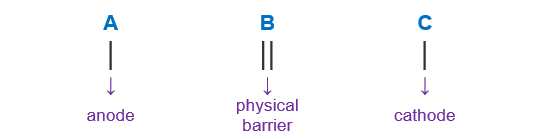# Problem: For the following electrochemical cell:Cu(s)/Cu^2+(aq,0.0155M//Ag+(aq,2.50M)/Ag(s)Write the net cell equation. Phases are optional. No not include concentrations.Calculate the following values at 25 °C using standard potentials as needed:Ecell(standard) = ____ vEcell = ____vΔ G°rxn standard= kJ/molΔ G°rxn = kJ/mol

###### FREE Expert Solution

When writing a cell notation, we use the following format – “as easy as ABCHence, the Cu/Cu+2 is the anode reaction while Ag+/Ag is the cathode reaction

(1) Calculating E°cell

$\overline{){\mathbf{E}}{{\mathbf{°}}}_{{\mathbf{cell}}}{\mathbf{=}}{\mathbf{E}}{{\mathbf{°}}}_{{\mathbf{cathode}}}{\mathbf{-}}{\mathbf{E}}{{\mathbf{°}}}_{{\mathbf{anode}}}}$

E°cell = 0.08 – (0.34)

cell = +0.46 V

(2) Calculating Ecell using Nernst equation:

Ecell = +0.53 V

91% (92 ratings)###### Problem Details

For the following electrochemical cell:

Cu(s)/Cu^2+(aq,0.0155M//Ag+(aq,2.50M)/Ag(s)

Write the net cell equation. Phases are optional. No not include concentrations.

Calculate the following values at 25 °C using standard potentials as needed:

Ecell(standard) = ____ v

Ecell = ____v

Δ G°rxn standard= kJ/mol

Δ G°rxn = kJ/mol